Earn 20 XP

## Functions

• This is something we have been indirectly using since the first module.
• print( ) and input( ) are some functions that you’ve already used. And these are inbuilt functions.
```1 ````print("hello world")`

```1 ````input("enter your age")`
• If you want, you can create your own function to get some desired output.
• Let's learn some in-built functions in the video mentioned below.

### Examples of in-built functions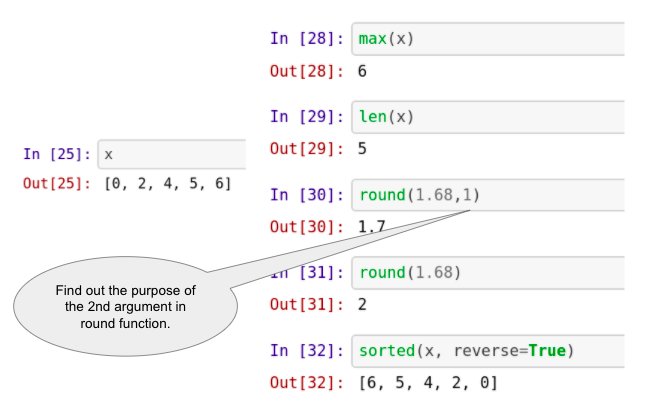## sum() and round()

### round()

• Round function in python rounds off the decimal value of a number to its nearest integer. For example: round(65.66) = 66.
• In addition, one can input the specified number of decimals to which the number should be rounded. For example, round(65.66, 1) = 65.7.
• Its syntax is:
```1 ````round(number, ndigits)`
• number - the number to be rounded.
• ndigits - number of decimal places up to which the given number is rounded; defaults to 0.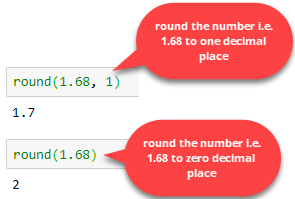### sum()

You can perform the addition of a collection of numbers using the sum() function in Python.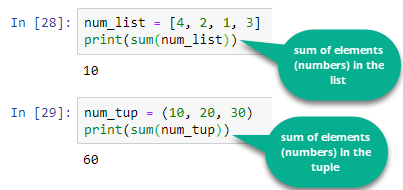## Defining Functions

• In Python, a function is defined using the def keyword.

• In the next tutorial, we will be learning:

• How to write a function
• How to call a function - Of course, if we have created a function, we need to use it by calling it, right? ;)
• We will also learn how to write a function that would convert bitcoin into USD :P
• Note: The tutor used some other user-friendly local Python IDE (similar to Colab or Jupyter notebook), so don't press the panic button looking at the new coding interface. You can comfortably run the same code on Colab/Jupyter notebook (or any python environment), and it will work.

## Methods

• In Python, everything is an object (a list, string, etc.).

• A method in Python is somewhat similar to a function. The only difference is that a method depends on the object while a function is independent of the object.

• Can you recall we had used a function len() in the earlier modules? Well, this is a function. len() can be used on lists, tuples, strings, dictionaries, etc., to get the number of elements in them. In comparison, append() is a method associated with lists only. You cannot use the append() method on any tuples, strings, or dictionaries. This append() is associated with list data type only.

• Objects have various methods associated with them (depending on the type of the object).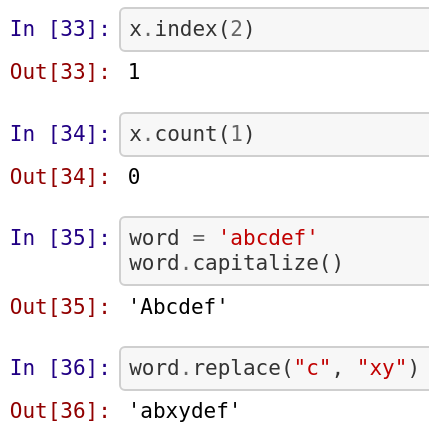## Functions vs. Methods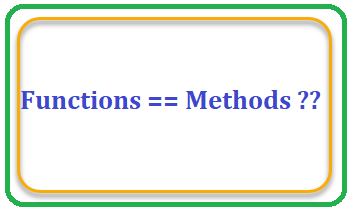• You might have a question: "Why on Earth do we have both functions and methods when they practically do the same thing?"

• Firstly, let's start with the obvious. There is a clear difference in the syntax:

• A function looks like this: function(something)
• And a method looks like this: something.method()
• Namely: a method always belongs to an object (e.g., in the x.index(2) method, .index() needed the x object to be applicable), while a function doesn't necessarily depend on a Python object.

• All methods are functions, but not all functions are methods!

• If this makes no sense to you (yet), don't you worry. I promise the idea will grow on you as you use Python more and more – especially when you start to define your functions and methods.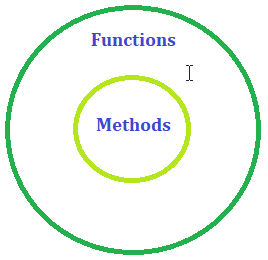## Packages & Modules

• In simple terms, Python packages are collections of multiple Python files.
• And these Python files are known as modules in Python.

### Need for Python Packages

• If we keep all of our code in the same file, it will result in:

• Huge code base: Ends up messy
• Lots of code you won't use
• Maintenance problem
• If we take an example of ourselves, we don't usually store all our files on our computer in the same location. We use a well-organized hierarchy of directories/folders for easier access.

• Similar files are kept in the same directory. For example, we may keep all the songs in the "music" directory. Analogous to this, Python has packages for directories and modules for files.

• As our application program grows larger with many modules, we place similar modules in one package and different modules in different packages. This makes a project (program) easy to manage and conceptually clear.

• Similarly, as a directory can contain subdirectories and files, a Python package can have sub-packages and modules.

• Packages are Directory/Folder of Python Scripts.

• Where each script is a module that performs a specific function.

• We can specify functions, methods, and types in a script.

• Thousands of packages are available in Python.

• For data science, the commonly used packages are:

• Numpy: Working with arrays
• Matplotlib: Data Visualisation
• Scikit-learn: ML

### Importing packages/modules

• A Python package can have sub-packages in it. Further, these sub-packages have some modules (i.e., Python files).

• Each module consists of some Python functions.

• We need to load the module in our working environment to use these functions.

• To load any package or module, we use the term import followed by module name or the package name.

• In the video, the tutor has loaded the 'math' module of Python and used functions like sqrt (to calculate the square root of a number), pow (to calculate the power of a number), etc.